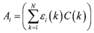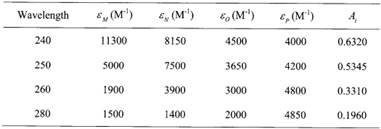Create an Account

Home / Questions / One of the most widely used applications of spectroscopy is for the quantitative determina...

One of the most widely used applications of spectroscopy is for the quantitative determination of the concentration of biological molecules in solution The absorbance of a solution Ai at wavelength

One of the most widely used applications of spectroscopy is for the quantitative determination of the concentration of biological molecules in solution. The absorbance of a solution, Ai, at wavelength i, is given by the sum of the product of e(k), the extinction coefficient/length of all components k (from 1 to N) obtained at the same wavelength and the concentrations of the components of the solution, C(k) (Tinoco et al., 2002).Consider a hypothetic experiment when a protein solution with four amino acids, M, N, O, and P, was measured using four different wavelengths, and the absorbance values were recorded. The extinction coefficients for the four amino acids for the four wavelengths are tabulated below. Estimate the concentration of the four amino acids in M. Compare your answers and the efficiency of solution using the Gauss Jordan method and the iterative Jacobi method.View less »

Jul 23 2020 View more View LessSubscribe To Get Solution